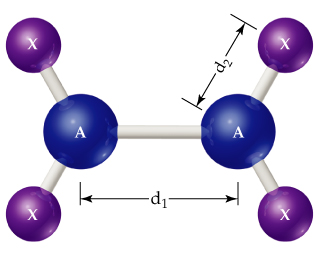# Problem: Consider the A2X4 molecule depicted below, where A and X are elements. The A- bond length in this molecule is exttip{d_{ m 1}}{d_1}, and the four A- bond lengths are each exttip{d_{ m 2}}{d_2}.In terms of exttip{d_{ m 1}}{d_1} and/or exttip{d_{ m 2}}{d_2}, how could you define the bonding atomic radius of atom X?

###### FREE Expert Solution
87% (427 ratings)
###### Problem Details

Consider the A2X4 molecule depicted below, where A and X are elements. The A- bond length in this molecule is , and the four A- bond lengths are each .In terms of and/or , how could you define the bonding atomic radius of atom X?

Frequently Asked Questions

What scientific concept do you need to know in order to solve this problem?

Our tutors have indicated that to solve this problem you will need to apply the Periodic Trend: Atomic Radius concept. You can view video lessons to learn Periodic Trend: Atomic Radius. Or if you need more Periodic Trend: Atomic Radius practice, you can also practice Periodic Trend: Atomic Radius practice problems.